# JEE Main 2020 Chemistry Paper With Solutions Sep 2 Shift 1

JEE Main 2020 Chemistry Paper with Solutions for September 2nd - Shift 1 is a perfect resource to score high marks in the upcoming JEE exams. These question papers will aid them during their preparation for the exams. The solutions uploaded at BYJU’S will enable the students to obtain a fair idea about the blueprint of the question paper pattern. Practising these questions will help them understand the amount of preparation required to face the examination.

### September 2 Shift 1 - Chemistry

Question 1.The increasing order of the following compounds towards HCN addition is: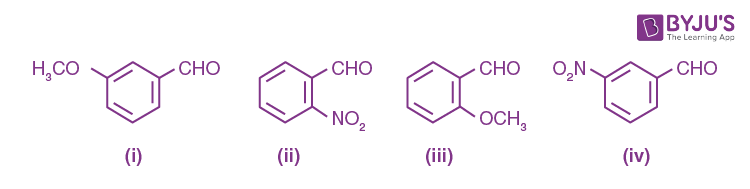1. a) (iii) < (i) < (iv) < (ii)
2. b) (iii) < (iv) < (i) < (ii)
3. c) (i) < (iii) < (iv) < (ii)
4. d) (iii) < (iv) < (ii) < (i)

Solution:

1. In HCN, CN acts as a nucleophile, attack first that –CHO group which has a maximum positive charge. The magnitude of the (+ve) charge increases by –M and –I group. So reactivity order will be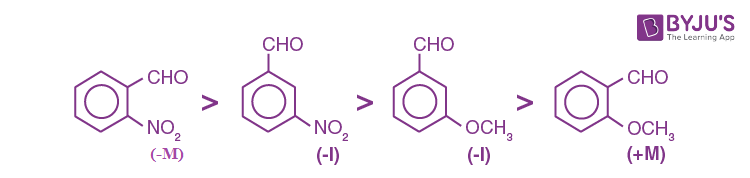So, option (1) is the correct answer.

Question 2. Which of the following is used for the preparation of colloids?

1. a) Van Arkel Method
2. b) Ostwald Process
3. c) Mond Process
4. d) Bredig’s Arc Method

Solution:

1. Bredig’s Arc method

Chapter name surface chemistry

Question 3. An open beaker of water in equilibrium with water vapour is in a sealed container. When a few grams of glucose are added to the beaker of water, the rate at which water molecules:

1. a) leaves the vapour increases
2. b) leaves the solution increases
3. c) leaves the vapour decreases
4. d) leaves the solution decreases

Solution:

1.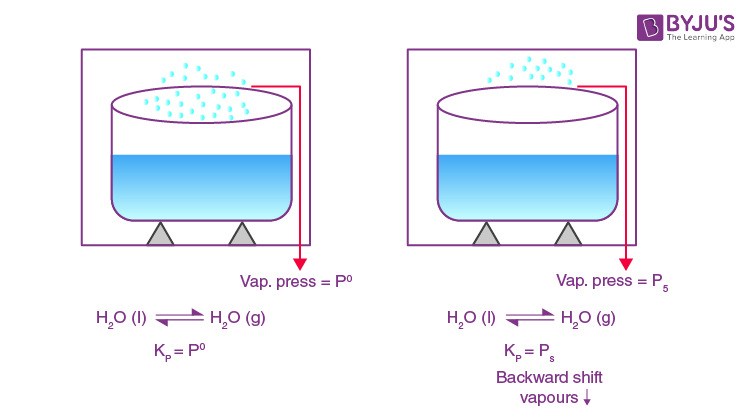Hence, Rate at which water molecules leave the vapour increases.

Question 4. For octahedral Mn(II) and tetrahedral Ni(II) complexes, consider the following statements:

(I) both the complexes can be high spin.

(II) Ni(II) complexes can very rarely be low spin.

(III) with strong field ligands, Mn(II) complexes can be low spin.

(IV) the aqueous solution of Mn(II) ions is yellow in colour.

The correct statements are:

1. a) (I), (III) and (IV) only
2. b) (I), (II) and (III) only
3. c) (II), (III) and (IV) only
4. d) (I) and (II) only

Solution:

1. Mn2+ [Ar]3d5 it can form low spin as well as high spin complex depending upon nature of ligand same of Ni2+ ion with coordination no 4. It can be dsp2 or sp3 i:e low spin or high spin depending on the open nature of the ligand.

Question 5. The statement that is not true about ozone is:

1. a) in the stratosphere, it forms a protective shield against UV radiation.
2. b) in the atmosphere, it is depleted by CFCs.
3. c) in the stratosphere, CFCs release chlorine-free radicals (Cl) which reacts with O3 to give chlorine dioxide radicals.
4. d) it is a toxic gas and its reaction with NO gives NO2.

Solution:

1.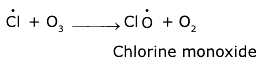Question 6. Consider the following reactions: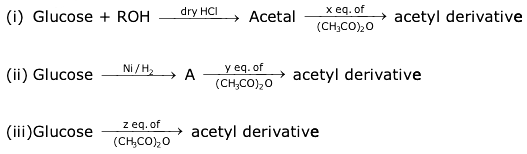'x', 'y' and 'z' in these reactions are respectively.

1. a) 4, 5 & 5
2. b) 5, 4 & 5
3. c) 5, 6 & 5
4. d) 4, 6 & 5

Solution:

1. (CH3CO)2O reacts with –OH group to form acetyl derivative, so as the no. of –OH group no. of eq. of (CH3CO)2O will be used

So, x = 4 y = 6 z = 5

So, option (4) will be the correct answer.

Question 7. The IUPAC name for the following compound is: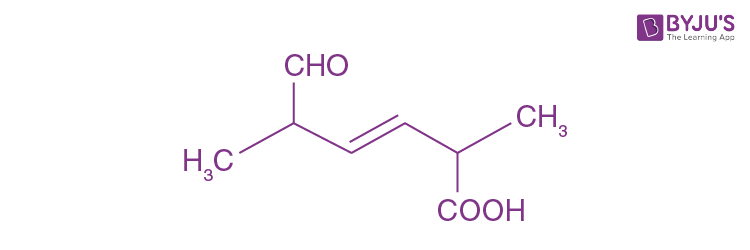1. a) 2,5-dimethyl-5-carboxy-hex-3-enal
2. b) 2,5-dimethyl-6-oxo-hex-3-enoic acid
3. c) 6-formyl-2-methyl-hex-3-enoic acid
4. d) 2,5-dimethyl-6-carboxy-hex-3-enal

Solution:

1.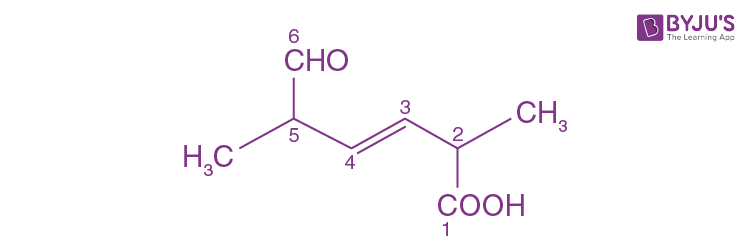2,5–Dimethyl–6–oxohex–3–enoic acid

Question 8. For the following Assertion and Reason, the correct option is Assertion (A): When Cu (II) and Sulphide ions are mixed, they react together extremely quickly to give a solid.

Reason (R): The equilibrium constant of Cu2+ (aq) + S2– (aq) ⇌ CuS (s) is high because the solubility product is low.

1. a) (A) is false and (R) is true.
2. b) Both (A) and (R) are false.
3. c) Both (A) and (R) are true but (R) is not the explanation for (A).
4. d) Both (A) and (R) are true but (R) is the explanation for (A).

Solution:

1. (A) is (B) true & (R) is the correct explanation of (A)

Question 9. Which one of the following graphs is not correct for an ideal gas?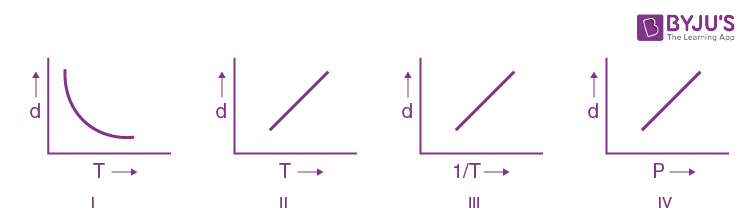d = Density, P = Pressure, T = Temperature

The correct statements are:

1. a) (i)
2. b) (iv)
3. c) (iii)
4. d) (ii)

Solution:

1. d = [P × M] / RT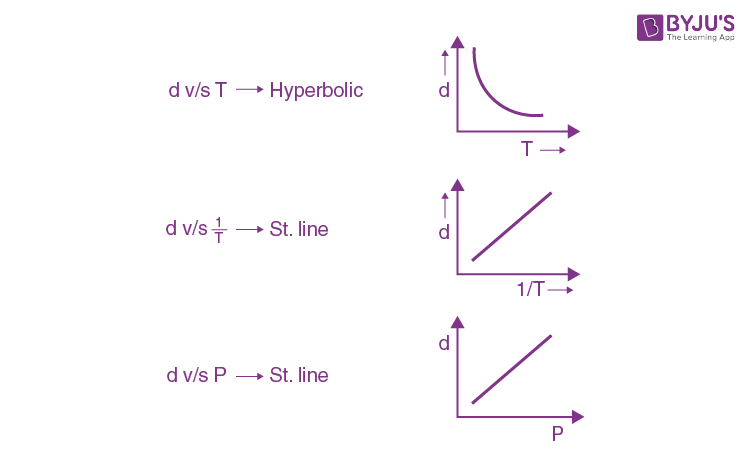∴ II’ Graph is incorrect.

Question 10. While titrating dilute HCl solution with aqueous NaOH, which of the following will not be required?

1. a) Bunsen burner and measuring cylinder
2. b) Burette and porcelain tile
3. c) Clamp and phenolphthalein
4. d) Pipette and distilled water

Solution:

1. Bunsen Burner & measuring cylinder are not Required. As titration is already on the exothermic process

Question 11.In Carius' method of estimation of halogen, 0.172 g of an organic compound showed the presence of 0.08 g of bromine. Which of these is the correct structure of the compound?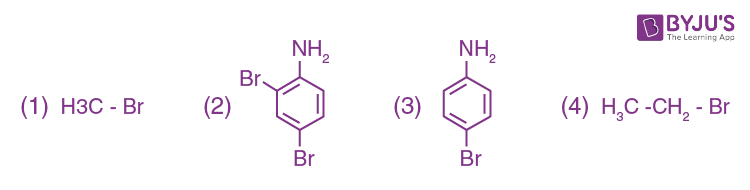Solution:

1. Carius method

mass % of ‘Br’ = [0.08 / 0.172] * 100 = (8000 / 172) = 46.51%

option (1) mass % = (80 / 95) * 100 =

(2) mass % = (2 * 80 * 100) / 252

(3) mass % = (1 * 80 * 100) / (80 + 72 + 6 + 14) = [8000 / 172] %

(4) mass % = [(1 * 80 * 100) / 109] %

Option (3) matches with the given mass percentage value

Question 12. On heating compound (A) gives a gas (B) which is a constituent of air. This gas when treated with H2 in the presence of a catalyst gives another gas (C) which is basic in nature. (A) should not be:

1. a) (NH4)2Cr2O7
2. b) NaN3
3. c) NH4NO2
4. d) Pb(NO3)2

Solution:

1. The gas (B) is N2 which is found in the air.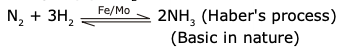NH3 + H2O → NH4OH (weak base)

(NH4)2Cr2O7 → N2 + Cr2O3 + H2O

NaN3 → N2 + Na

NH4NO2 → N2 + H2O

Pb(NO3)2 → PbO + NO2 + O2

Question 13. The major product in the following reaction is: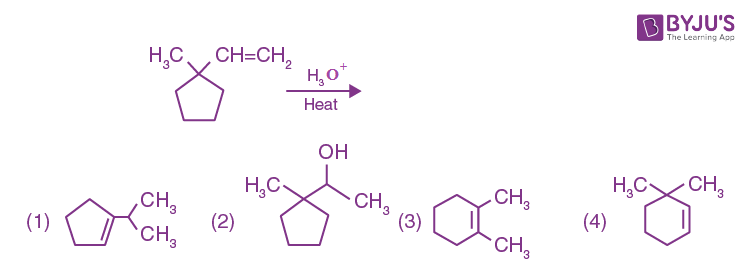Solution:

1.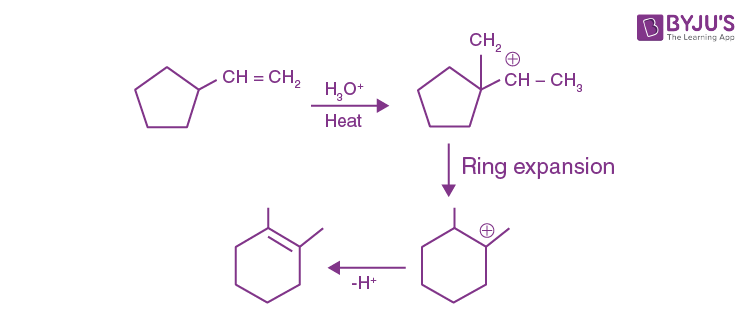Option (3) is the correct answer.

Question 14. In general, the property (magnitudes only) that shows an opposite trend in comparison to other properties across a period is:

1. a) Ionization enthalpy
2. b) Electronegativity
4. d) Electron gain enthalpy

Solution:

1. Ionisation energy, electronegativity & electron gain enthalpy increase across a period but atomic radius decreases.

Question 15. The figure that is not a direct manifestation of the quantum nature of atoms is: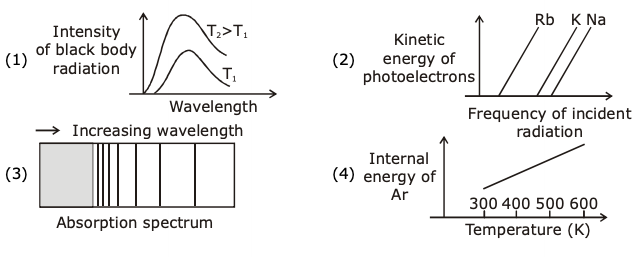Solution:

1. The internal energy of ‘Ar’ or any gas, has nothing to do with Quantum nature of atom hence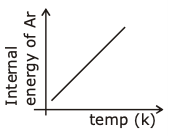Question 16. The major aromatic product C in the following reaction sequence will be: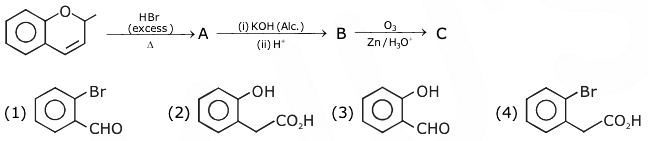Solution:

1.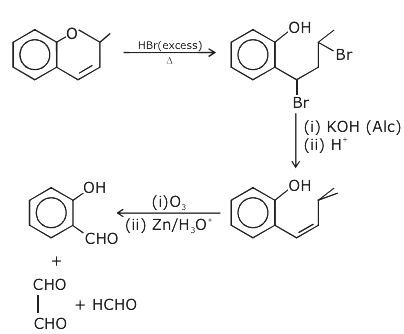Question 17. Consider that a d6 metal ion (M2+) forms a complex with aqua ligands, and the spin only magnetic moment of the complex is 4.90 BM. The geometry and the crystal field stabilization energy of the complex is:

1. a) tetrahedral and –0.6Δt
2. b) tetrahedral and –1.6Δt + 1P
3. c) octahedral and –1.6Δ0
4. d) octahedral and –2.4Δ0 + 2P

Solution:

1. Heat of combustion ∝ 1/ stability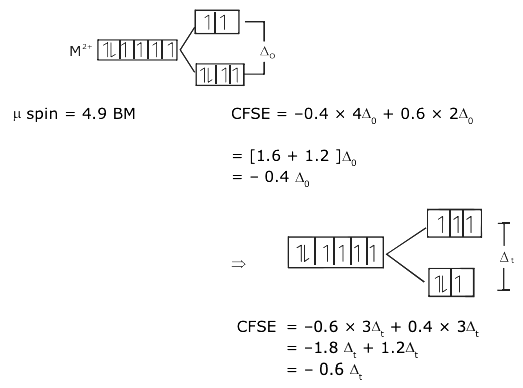Question 18. If AB4 molecule is a polar molecule, a possible geometry of AB4 is:

1. a) Square planar
2. b) Tetrahedral
3. c) Square pyramidal
4. d) Rectangular planar

Solution:

1. Incorrect question Option 1 is more appropriate with respect to the given option (Chemical bonding)

(Options are incorrect)

Question 19. Which of the following compounds will show retention in the configuration on nucleophilic substitution by OH ion?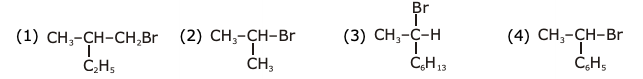Solution:

1. In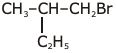attack of OH is not on chiral carbon, it is adjacent to chiral carbon, so the configuration of chiral carbon remains constant.

Question 20. The metal mainly used in devising photoelectric cells is:

1. a) Li
2. b) Cs
3. c) Rb
4. d) Na

Solution:

1. ‘Cs’ is used in the photoelectric cell as its ionisation energy is lowest.

Question 21. The mass of gas adsorbed, x, per unit mass of adsorbate, m, was measured at various pressures, p. A graph between log [x / m] and log p gives a straight line with slope equal to 2 and the intercept equal to 0.4771. The value of [x / m] at a pressure of 4 atm is: (Given log 3 = 0.4771)

Solution:

1. (x / m) = KP1/n

log (x / m) = log (k) + (1 / n) log (p)

y = c + mx

Intercept C = logk = 0.4771

Slope = 1 / n = 2, k = 3

(x / m) = k (P)1/n at P = 4 atm

= 3 (4)2

(x / m) = 3 * 16 = 48 Answer

Question 22.The Gibbs energy change (in J) for the given reaction at [Cu2+] = [Sn2+] = 1 M and 298 K is:

Cu(s) + Sn2+ (aq.) → Cu2+(aq.) + Sn(s)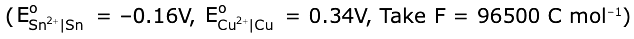Solution:

1. Cu(s) + Sn+2(aq) ⇋ Cu+2 (aq) + Sn(s)

cell = –0.16 – 0.34

= –0.50

ΔGº = –nF Eºcell

= – 2 × 96500 × (–0.5)

= +96500

ΔG = Gº + RT nQ

= 96500 + (25 / 3) × 298 × 2.303 log (1)

ΔG = 96500 Joules

Question 23. The internal energy change (in J) when 90 g of water undergoes complete evaporation at 100º C is __________.

(Given: ΔHvap for water at 373 K = 41 kJ/mol, R = 8.314 JK–1 mol–1)

Solution:

1. H2O (l) → H2O (g)

ΔEvap = ΔHvap - Δng RT

= 41000 × 5 – 5 × 8.314 × 373

= 189494.39

Question 24.The oxidation states of iron atoms in compounds (A), (B) and (C), respectively, are x, y and z. The sum of x, y and z is _____.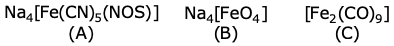Solution:

1. Na4 [Fe+2(CN)5 (NOS)]

Na4 [Fe+4O4]

[Fe20 (CO)9]

Question 25. The number of chiral carbons present in the molecule given below is ________.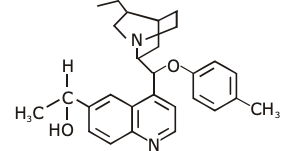Solution:

1.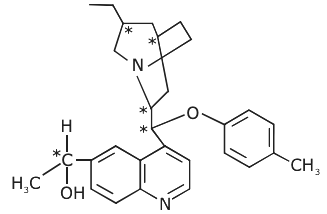Total chiral carbon = 5

### JEE Main 2020 Chemistry Paper With Solutions Sep 2 Shift 1